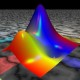Ask Your Question

# DeprecationWarning: Substitution using function-call syntax and unnamed arguments

Good morning,

I am trying to fix Deprecation Warning in my interact but I don't really know how to change the syntax to fix this warning. It says:

:16: DeprecationWarning: Substitution using function-call syntax and unnamed arguments is deprecated and will be removed from a future release of Sage; you can use named arguments instead, like EXPR(x=..., y=...)
:22: DeprecationWarning: Substitution using function-call syntax and unnamed arguments is deprecated and will be removed from a future release of Sage; you can use named arguments instead, like EXPR(x=..., y=...)
DeprecationWarning: Substitution using function-call syntax and unnamed arguments is deprecated and will be removed from a future release of Sage; you can use named arguments instead, like EXPR(x=..., y=...)
self._f(**dict([(k, self._last_vals[k]) for k in self._args]))


Could anyone help me?

The original is here:

@interact
def aproximace_delky_krivky(funkce = input_box(default = x*sin(x^2) + 7/2, type = SR),
dolni_mez_intervalu = input_box(default = 0, type = SR, label="dolní mez intervalu"),
horni_mez_intervalu = input_box(default = 4, type = SR, label="horní mez intervalu"),
pocet_deleni = slider(1, 100, 1, default=6, label="počet dělicích bodů")):

a = dolni_mez_intervalu
b = horni_mez_intervalu
t = var('t')
f = funkce
dx = RR(b-a)/RR(pocet_deleni)

xs = []
ys = []
for q in range(pocet_deleni):
x = q*dx + dx + a
xs.append(x)
ys = [f(x) for x in xs]

xz = []
yz = []
for q in range(pocet_deleni):
t = q*dx + a
xz.append(t)
yz = [f(t) for t in xz]

rects = Graphics()
for q in range(pocet_deleni):
x = xs[q]
y = ys[q]
g = xz[q]
h = yz[q]
rects += point((x, y), color="blue", pointsize=20)
rects += point((a, f(a)), color="blue", pointsize=20)
rects += line([(x, y), (g, h)], color="blue", thickness=2)

show(plot(f, (x, a-0.5, b+0.5), color="red") + rects)

priblizna_hodnota = sum([ sqrt((xz[q]-xs[q])^2 + (yz[q]-ys[q])^2) for q in range(pocet_deleni)])

show(N(priblizna_hodnota))

edit retag close merge delete

## 1 Answer

Sort by » oldest newest most votedThe problem comes from the fact that funkce is a symbolic expression and so it is f. Since f is not a symbolic callable function, you can not use the notation f(x). You have to replace x by the corresponding value, writing either f.subs(x=value) or f(x=value). So a simple solution is to replace

ys = [f(x) for x in xs]


and

yz = [f(t) for t in xz]


by

ys = [f(x=x) for x in xs]


and

yz = [f(x=t) for t in xz]


Hope this helps.

more

## Comments

@Adam_37_W - ideally, accept the answer by clicking the check mark at its top left. This marks the question as solved in the list of questions.

## Your Answer

Please start posting anonymously - your entry will be published after you log in or create a new account.

Add Answer

## Stats

Asked: 2021-02-03 14:12:05 +0200

Seen: 66 times

Last updated: Feb 03